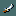## FANDOM

2,034 Pages

)Name silver saber
Appearance silver saber
Damage vs. small 1d8+(1d20)
Damage vs. large 1d8+(1d20)
To-hit bonus +0
Weapon skill saber
Size one-handed
Cost 75 zm
(+10/positive
enchant)
Weight 40
Material silver

The silver saber is a silver sword, commonly used as a secondary weapon in #twoweaponing, as it gives silver damage against lycanthropes, demons, vampires, minor demons (except tengu), and shades, in addition to being a respectable weapon in its own right.

## GenerationEdit

They are randomly generated like any other weapon, but the watch captain in Minetown has a 50% chance of being generated with one, as does any Captain. If Fort Ludios exists, you are extremely likely to find a saber there.

## Average damage calculationEdit

We assume the player has expert skill in saber, which gives a +2 damage bonus. A blessed weapon deals 1d4 extra damage against demons and undead, and being of silver, an added 1d20 to silver-hating monsters. The worst case scenario is against a non-undead, non-demon, non-silver-hating monster. The best case scenario is against a undead/demon silver-hating monster.

Weapon Against regular small monsters Against regular large monsters Worst case scenario Best case scenario
Blessed silver saber+0 $\frac{1+8}{2}+2=\bold{6.5}$ $\frac{1+8}{2}+2=\bold{6.5}$ $\frac{1+8}{2}+2=\bold{6.5}$ $\frac{1+8}{2}+\frac{1+20}{2}+\frac{1+4}{2}+2=\bold{19.5}$
Blessed silver saber +7 $\frac{1+8}{2}+2+7=\bold{13.5}$ $\frac{1+8}{2}+2+7=\bold{13.5}$ $\frac{1+8}{2}+2+7=\bold{13.5}$ $\frac{1+8}{2}+\frac{1+20}{2}+\frac{1+4}{2}+2+7=\bold{26.5}$
Blessed silver saber +9 $\frac{1+8}{2}+2+9=\bold{15.5}$ $\frac{1+8}{2}+2+9=\bold{15.5}$ $\frac{1+8}{2}+2+9=\bold{15.5}$ $\frac{1+8}{2}+\frac{1+20}{2}+\frac{1+4}{2}+2+9=\bold{28.5}$

Despite the inclusion in the above table, attempting to enchant a silver saber to +9 is generally a very bad idea, as they're quite rare.

## SLASH'EMEdit

SLASH'EM adds a new saber weapon: the rapier. A +1 rapier is guaranteed to appear in Grund's Stronghold.

## Saber skillEdit

Saber
Max Role
Basic
Skilled
Expert

The silver saber is the only weapon to use the saber skill. Artifact sabers are Grayswandir and Werebane.